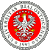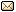XXXIX Workshop on Geometric Methods in Physics 27.06-3.07.2021 IX School on Geometry and Physics 21-25.06.2021
 Home First Announcement Travel and local information Lecturers (WGMP) Lecturers (SGP) Registration Photos Proceedings Previous Workshops Email

## Symplectic reduction for differential equations degenerating on the boundary

We study the geometry underlying semiclassical asymptotic solutions for a class of (pseudo)differential equations degenerating on the boundary $\partial X$ of a smooth compact manifold $X$. We use the fact that $X$ can be represented as the quotient of a closed smooth manifold $M$ by a semi-free circle action $\tau$, $\partial X$ being an isomorphic projection of the fixed-point set of $\tau$, and define the phase space $\Phi$ as the symplectic reduction of $T^*M$ with respect to the circle action $\hat\tau$ induced by $\tau$. Although the manifold $\Phi$ (equipped with the natural projection onto $X$) is isomorphic to $T^*X$ over the interior of $X$, it fails to be so in a neighborhood of the boundary (where it is not even a locally trivial bundle). This prevents one from using the standard procedure (Maslov’s canonical operator) for constructing asymptotic solutions $u$ associated with Lagrangian submanifolds $\Lambda\subset\Phi$, but the difficulty can be sidestepped if we not ice that $\Lambda$ can be viewed as the quotient of a $\hat\tau$-invariant Lagrangian manifold $L\subset T^*M$ and $u$ can be interpreted as a $\tau$-equivariant asymptotic solution of an appropriate lift of the original equation to $M$. Accordingly, $u$ can be written in terms of Maslov’s canonical operator on $L$, and it remains to represent the resulting expression via $\Phi$, $\Lambda$, and other objects related to $X$ alone. When doing so, we not only obtain a concise definitive formula for the canonical operator on $\Lambda$ but also clarify the nature of the Maslov index on Lagrangian submanifolds of $\Phi$. Finally, we present some examples related to shallow water equations. The talk is based on joint work with S.Yu. Dobrokhotov. The research was supported by the Russian Science Foundation under grant no. 16-11-10282.

 Event sponsored by: University of BialystokWebpage by: Tomasz Golinski